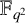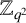Hostname: page-component-546b4f848f-gfk6d Total loading time: 0 Render date: 2023-06-03T18:39:59.148Z Has data issue: false Feature Flags: { "useRatesEcommerce": true } hasContentIssue false

## Abstract

A Sidon set is a subset of an Abelian group with the property that the sums of two distinct elements are distinct. We relate the Sidon sets constructed by Bose to affine subspaces of$\mathbb {F} _ {q ^ 2}$ of dimension one. We define Sidon arrays which are combinatorial objects giving a partition of the group$\mathbb {Z}_{q ^ 2}$ as a union of Sidon sets. We also use linear recurring sequences to quickly obtain Bose-type Sidon sets without the need to use the discrete logarithm.

## MSC classification

Type
Research Article
Information
Bulletin of the Australian Mathematical Society , December 2022 , pp. 376 - 384
© The Author(s), 2022. Published by Cambridge University Press on behalf of Australian Mathematical Publishing Association Inc.

## Access options

Get access to the full version of this content by using one of the access options below. (Log in options will check for institutional or personal access. Content may require purchase if you do not have access.)

## References

Bose, R. C., ‘An affine analogue of Singer’s theorem’, J. Indian Math. Soc. (N.S.) 6 (1942), 115.Google Scholar
Caicedo, Y., Martos, C. A. and Trujillo, C. A., ‘$g$ -Golomb rulers’, Rev. Integr. 33 (2015), 161172.Google Scholar
Davydov, A. A., Faina, G., Giulietti, M., Marcugini, S. and Pambianco, F., ‘On constructions and parameters of symmetric configurations${v}_k$ ’, Des. Codes Cryptogr. 80 (2016), 125147.CrossRefGoogle Scholar
Ganley, M. J., ‘Direct product difference sets’, J. Combin. Theory Ser. A 23 (1977), 321332.CrossRefGoogle Scholar
Gilberto, G. P., Alberto, T. S. C. and Velasquez, J. M., ‘Construccion de conjuntos B h módulo$m$ y particiones’, Mat. E. Univ. XIV (2006), 6570.Google Scholar
Lidl, R. and Niederreiter, H., Introduction to Finite Fields and Their Applications (Cambridge University Press, New York, 2000).Google Scholar
Lindström, B., ‘Finding finite B2-sequences faster’, Math. Comp. 67 (1998), 11731178.CrossRefGoogle Scholar
Martos Ojeda, C. A., Delgado Ordoñez, L. M. and Trujillo Solarte, C. A., ‘B h sets as a generalization of Golomb rulers’, IEEE Access 9 (2021), 118042118050.CrossRefGoogle Scholar
O’Bryant, K., ‘A complete annotated bibliography of work related to Sidon sequences’, Electron. J. Combin. DS11 (2004), 39 pages.Google Scholar
Roth, R. M., Raviv, N. and Tamo, I., ‘Construction of Sidon spaces with applications to coding’, IEEE Trans. Inform. Theory 64(6) (2018), 44124422.CrossRefGoogle Scholar
Xiu, B., Fan, C. and Liang, M., ‘On disjoint Golomb rulers’, Preprint, 2014, arXiv:1405.4535.Google Scholar